# 线性表、顺序表和链表，你还分不清？

2021/06/29 11:27

## 前言

• 线性表：逻辑结构， 就是对外暴露数据之间的关系，不关心底层如何实现，数据结构的逻辑结构大分类就是线性结构和非线性结构而顺序表、链表都是一种线性表。
• 顺序表、链表：物理结构，他是实现一个结构实际物理地址上的结构。比如顺序表就是用数组实现。而链表用指针完成主要工作。不同的结构在不同的场景有不同的区别。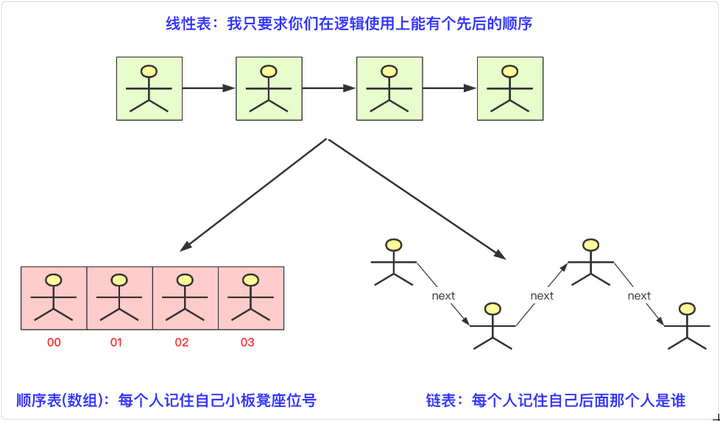## 线性表基本架构

package LinerList;
public interface ListInterface<T> {
void Init(int initsize);//初始化表
int length();
boolean isEmpty();//是否为空
int ElemIndex(T t);//找到编号
T getElem(int index) throws Exception;//根据index获取数据
void add(int index,T t) throws Exception;//根据index插入数据
void delete(int index) throws Exception;
void set(int index,T t) throws Exception;
String toString();//转成String输出
}

## 顺序表

### 插入操作

(1)从后(最后一个有数据位)向前到index依次后移一位，腾出index位置的空间

(2)将待插入数据赋值到index位置上，完成插入操作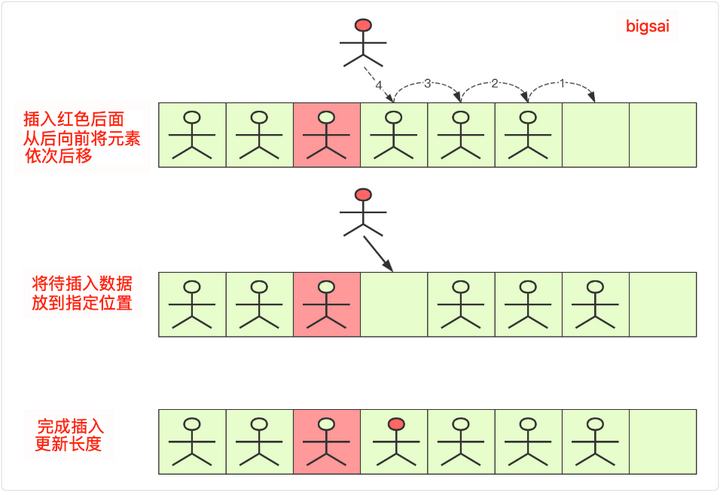### 删除操作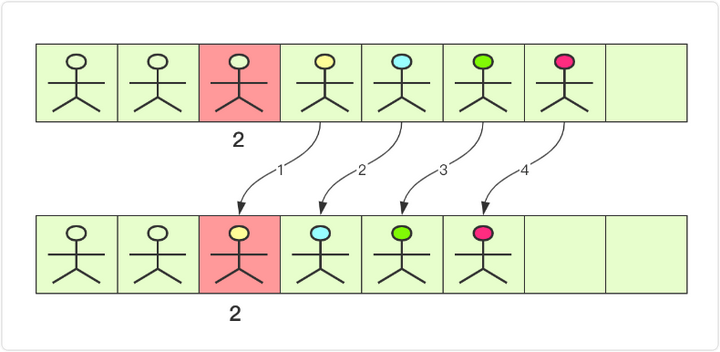### 代码实现

package LinerList;

public class seqlist<T> implements ListInterface<T> {
private Object[] date;//数组存放数据
private int lenth;
public seqlist() {//初始大小默认为10
Init(10);
}

public void Init(int initsize) {//初始化
this.date=new Object[initsize];
lenth=0;
}
public int length() {
return this.lenth;
}

public boolean isEmpty() {//是否为空
if(this.lenth==0)
return true;
return false;
}

/*
* * @param t
* 返回相等结果，为-1为false
*/
public int ElemIndex(T t) {
// TODO Auto-generated method stub
for(int i=0;i<date.length;i++)
{
if(date[i].equals(t))
{
return i;
}
}
return -1;
}

/*
*获得第几个元素
*/
public T getElem(int index) throws Exception {
// TODO Auto-generated method stub
if(index<0||index>lenth-1)
throw new Exception("数值越界");
return (T) date[index];
}

public void add(T t) throws Exception {//尾部插入
}

/*
*根据编号插入
*/
public void add(int index, T t) throws Exception {
if(index<0||index>lenth)
throw new Exception("数值越界");
if (lenth==date.length)//扩容
{
Object newdate[]= new Object[lenth*2];
for(int i=0;i<lenth;i++)
{
newdate[i]=date[i];
}
date=newdate;
}
for(int i=lenth-1;i>=index;i--)//后面元素后移动
{
date[i+1]=date[i];
}
date[index]=t;//插入元素
lenth++;//顺序表长度+1

}

public void delete(int index) throws Exception {
if(index<0||index>lenth-1)
throw new Exception("数值越界");
for(int i=index;i<lenth;i++)//index之后元素前移动
{
date[i]=date[i+1];
}
lenth--;//长度-1
}

@Override
public void set(int index, T t) throws Exception {
if(index<0||index>lenth-1)
throw new Exception("数值越界");
date[index]=t;
}
public String  toString() {
String vaString="";
for(int i=0;i<lenth;i++)
{
vaString+=date[i].toString()+" ";
}
return vaString;

}
}

## 链表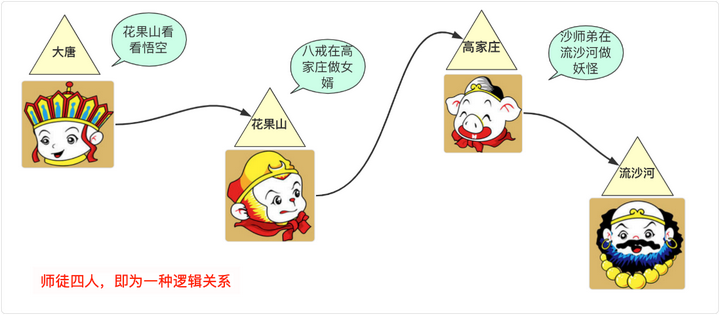### 基本结构

class node<T>{
T data;//节点的结果
node next;//下一个连接的节点
public node(){}
public node(T data)
{
this.data=data;
}
public node(T data, node next) {
this.data = data;
this.next = next;
}
}

### 带头结点链表VS不带头结点链表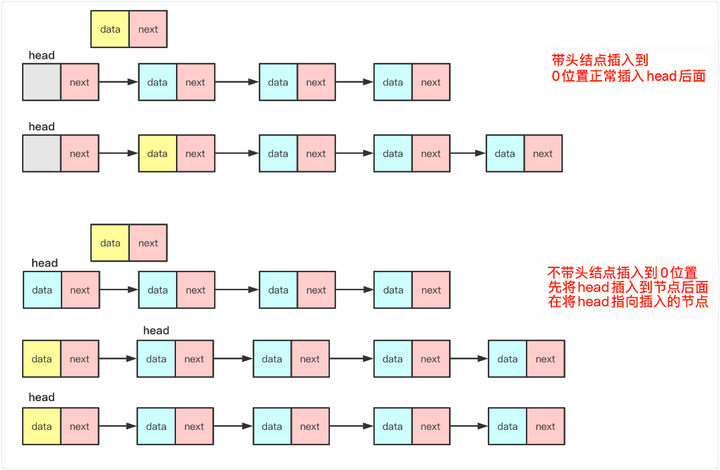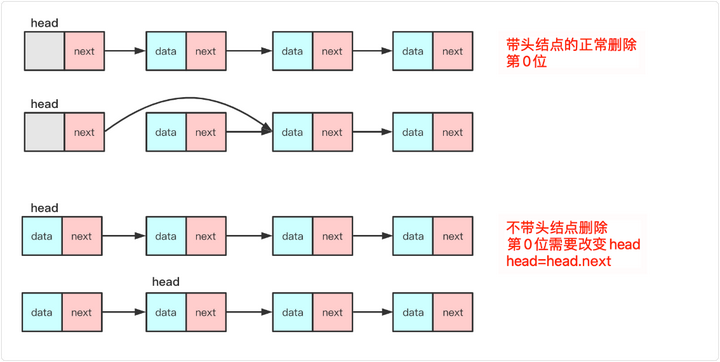### 带头指针VS带尾指针

**尾指针： **尾指针就是多一个tail节点的链表，尾指针的好处就是进行尾插入的时候可以直接插在尾指针的后面，然后再改变一下尾指针的顺序即可。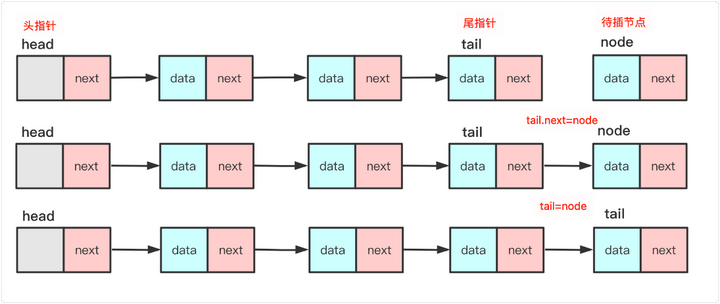### 插入操作

1. node.next=pre.next，将插入节点后面先与链表对应部分联系起来。此时node.next和pre.next一致。
2. pre.next=node 将node节点插入到链表中。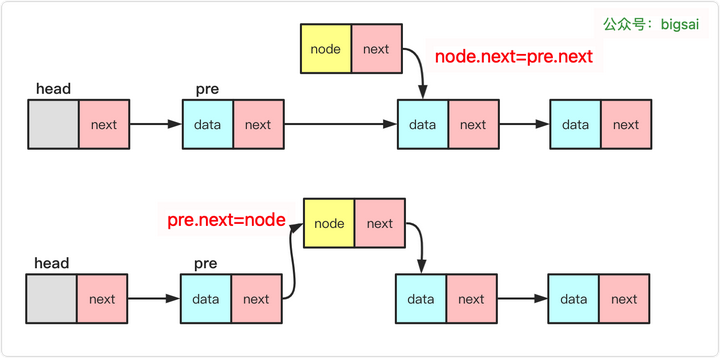### 删除操作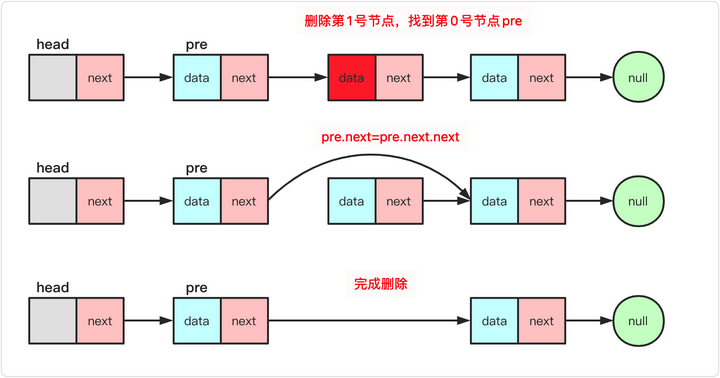### 代码实现

package LinerList;

class node<T>{
T data;//节点的结果
node next;//下一个连接的节点
public node(){}
public node(T data)
{
this.data=data;
}
public node(T data, node next) {
this.data = data;
this.next = next;
}

}

private int length;
length=0;
}
public void Init(int initsize) {

}

public int length() {
return this.length;
}
public boolean isEmpty() {
if(length==0)return true;
else return false;
}

/*
* 获取元素编号
*/
public int ElemIndex(T t) {
int index=0;
while(team.next!=null)
{
if(team.data.equals(t))
{
return index;
}
index++;
team=team.next;
}
return -1;//如果找不到
}

@Override
public T getElem(int index) throws Exception {
if(index<0||index>length-1)
{
throw new Exception("数值越界");
}
for(int i=0;i<index;i++)
{
team=team.next;
}
return (T) team.data;
}
public void add(T t) throws Exception {

}
//带头节点的插入，第一个和最后一个一样操作
public void add(int index, T value) throws Exception {
if(index<0||index>length)
{
throw new Exception("数值越界");
}
for(int i=0;i<index;i++)
{
team=team.next;
}
node<T>node =new node(value);//新建一个node
node.next=team.next;//指向index前位置的下一个指针
team.next=node;//自己变成index位置
length++;
}
@Override
public void delete(int index) throws Exception {
if(index<0||index>length-1)
{
throw new Exception("数值越界");
}
for(int i=0;i<index;i++)//标记team 前一个节点
{
team=team.next;
}
//team.next节点就是我们要删除的节点
team.next=team.next.next;
length--;
}

@Override
public void set(int index, T t) throws Exception {
// TODO Auto-generated method stub
if(index<0||index>length-1)
{
throw new Exception("数值越界");
}
for(int i=0;i<index;i++)
{
team=team.next;
}
team.data=t;//将数值赋值，其他不变

}

public String toString() {
String va="";
while(team!=null)
{
va+=team.data+" ";
team=team.next;
}
return va;
}

}

## 总结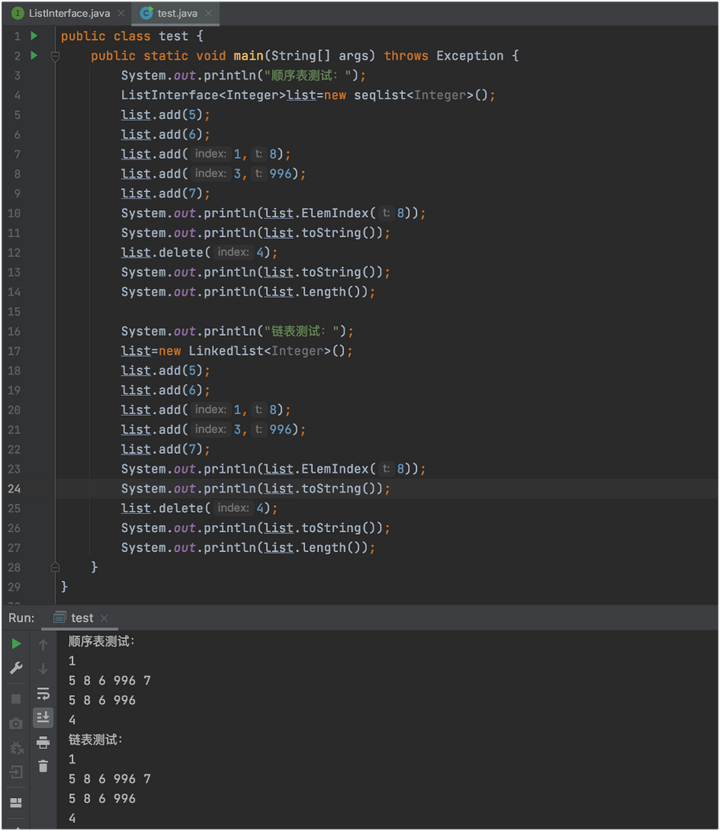0 评论
0 收藏
0